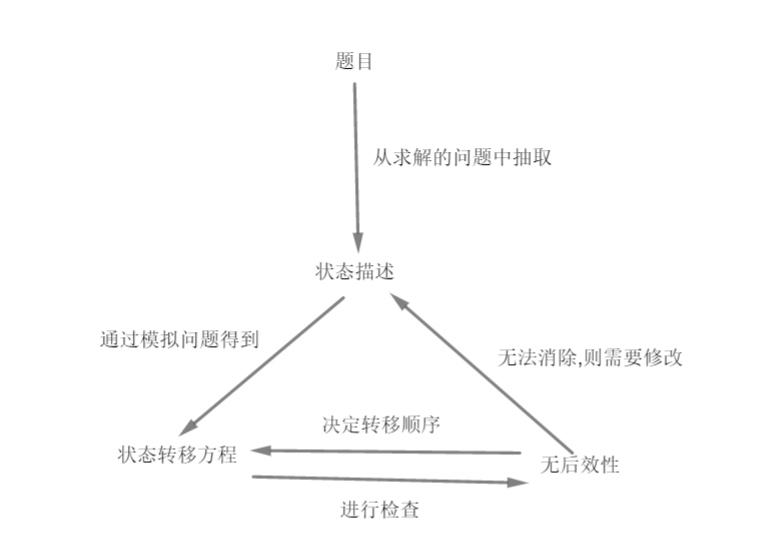# 上机题目题解

## 电力分配

n=int(input())
a=[0 for i in range(n)]
for i in range(n):
a[i]=int(input())
m=int(input())
b=[0 for i in range(m)]
for i in range(m):
b[i]=int(input())
b.sort()
ans=0
for i in b:
for j in range(n):
if (i>=a[j]):
ans+=1
a[j]=0x3f3f3f3f
break
print(ans)


## 我不是盘神

n=int(input())
a=[0 for i in range(n)]
for i in range(n):
a[i]=int(input())
a.sort()
up=n//3
ans=0
for i in range(up):
ans+=a[i]
print(ans)
for i in range(up):
print(a[i],end=' ')


## 装备选择

n=int(input())#下面会把这个问题转化为背包模型
w=[0 for i in range(n)]#物品体积
c=[0 for i in range(n)]#物品价值
for i in range(n):
w[i],c[i]=map(int,input().split())
m=int(input())
f=[0 for i in range(m+100)]#用于dp的数组
p=[0 for i in range(m+100)]#储存选择情况的数组
for i in range(n):
for j in range(m,w[i]-1,-1):
if (f[j-w[i]]+c[i]>f[j]):
f[j]=f[j-w[i]]+c[i]#更新答案
p[j]=p[j-w[i]]|(1<<i)#更新状态
print(f[m])
cnt=0
ans=''
for i in range(n):
if (p[m]&(1<<i)):
cnt+=1
ans=ans+str(i)+' '
print(cnt,ans,sep='\n')


## 我不是盘神 Pro

n=int(input())
f=[[0 for i in range(2)] for i in range(n+10)]
for i in range(2,n+2):
x=int(input())
f[i]=min(f[i-2],f[i-1])
f[i]=min(f[i-1]+x,f[i-1]+x)
print(min(f[n+1],f[n+1]))


f[i]表示下载了前i个文件,最后一个文件是加速下载的,总共使用了多少时间;f[i]表示下载了前i个文件,最后一个文件是通过网盘下载的,总共使用了多少时间.为了防止溢出,下标从2开始.

f[i]=min(f[i-2],f[i-1])


f[i]=min(f[i-1]+x,f[i-1]+x)


# DP内容补充

## 01背包

dp[i]表示不超过i体积的背包可以装下的最大价值.

for i in range(n):
for j in range(w[i],m+1):
dp[j]=max(dp[j],dp[j-w[i]]+c[i])


for i in range(n):
for j in range(m+1,w[i]-1,-1):
dp[j]=max(dp[j],dp[j-w[i]]+c[i])## 多维DP

for i in range(n):
for j in range(m):
if (i>0):#边界情况判断
dp[i][j]+=dp[i-1][j]
if (j>0):#边界情况判断
dp[i][j]+=dp[i][j-1]